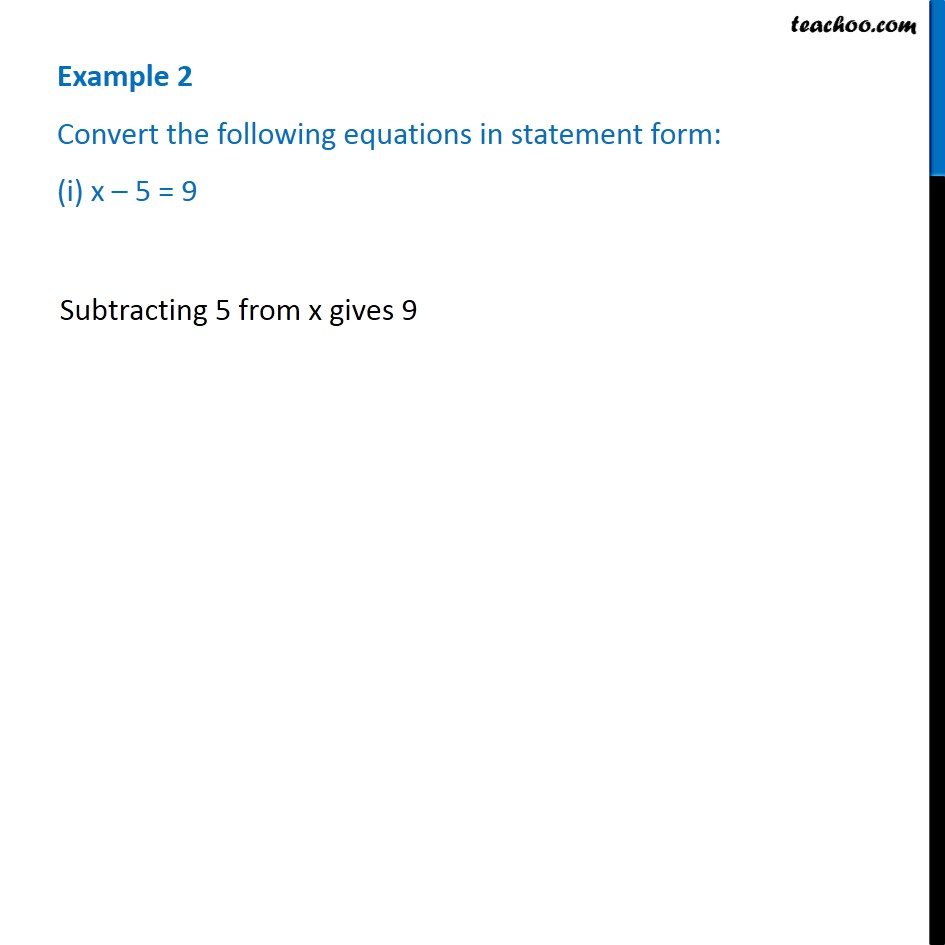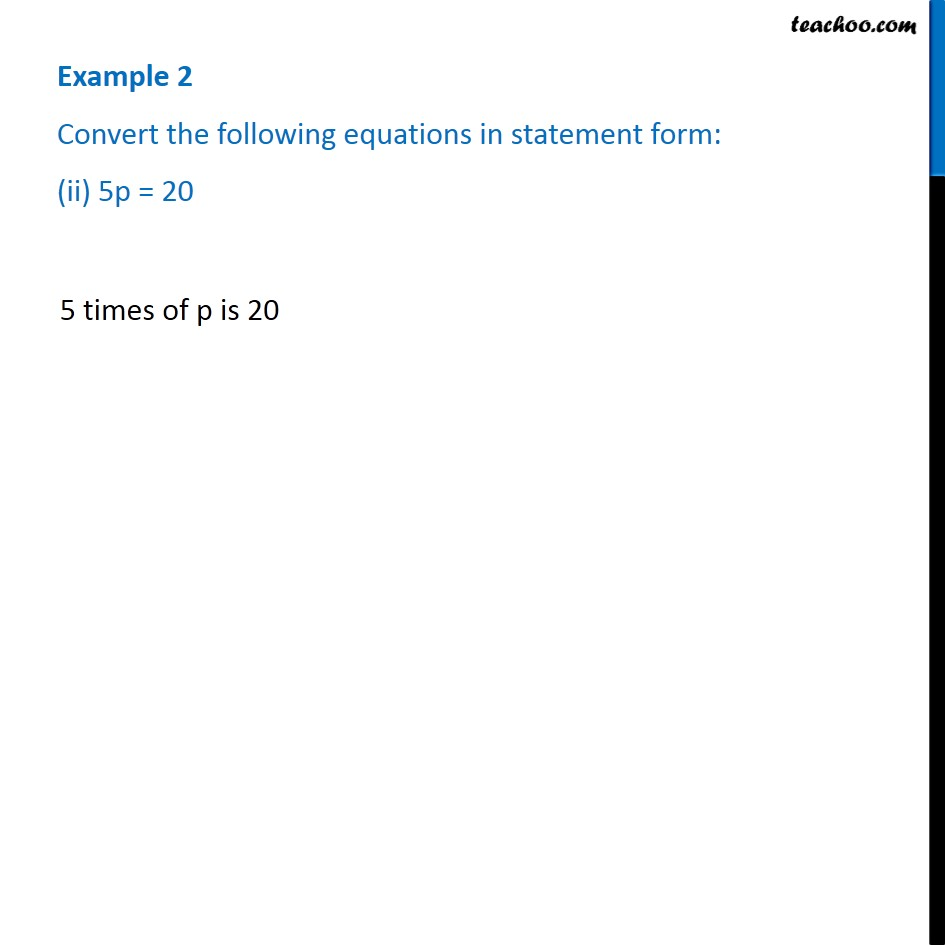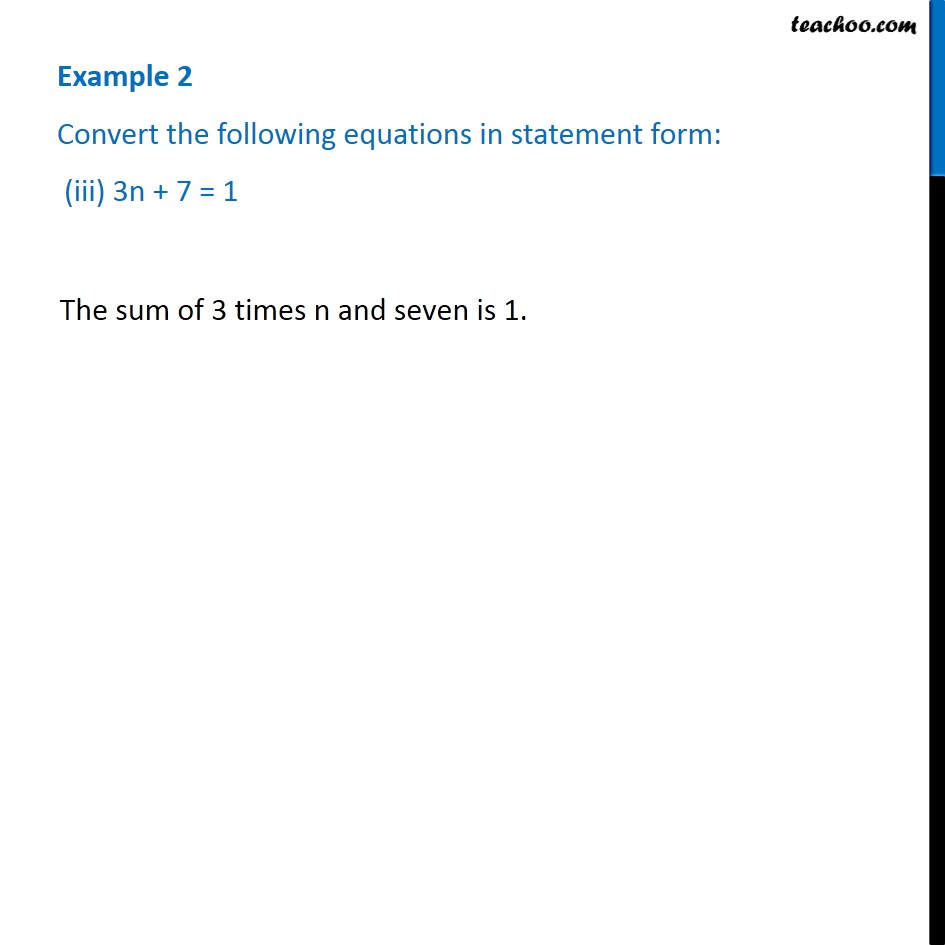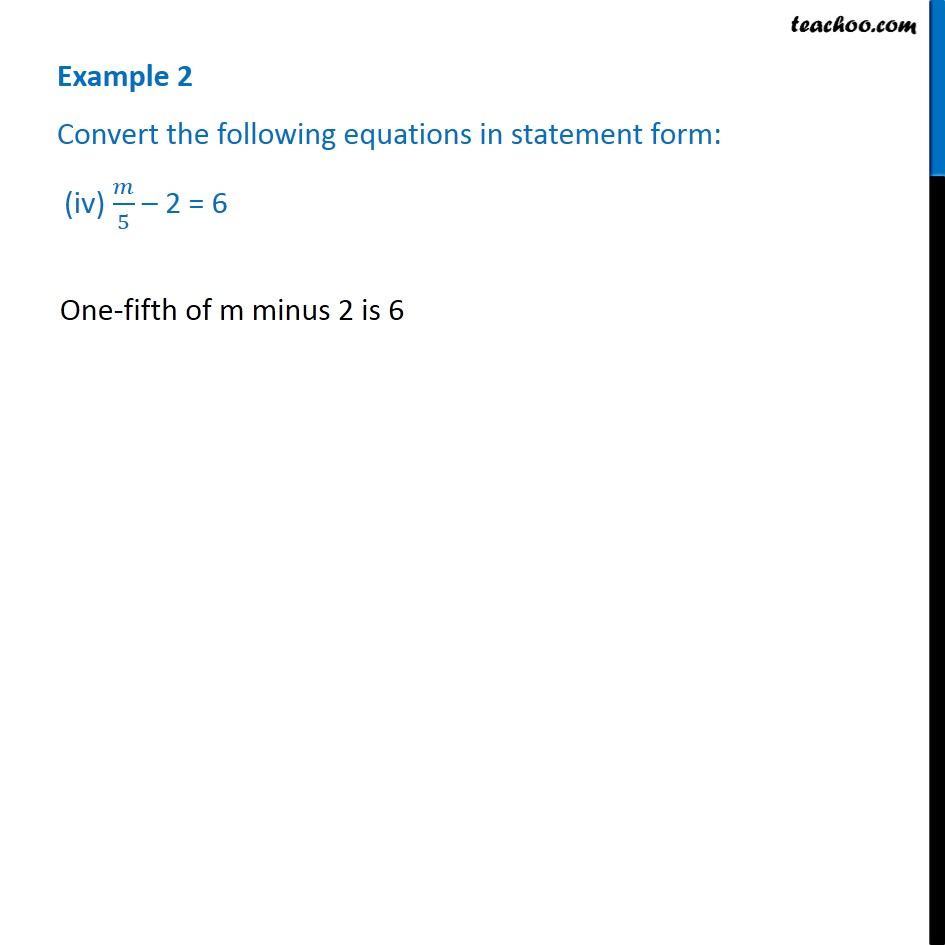1. Chapter 4 Class 7 Simple Equations
2. Concept wise
3. Writing equation to statements

Transcript

Example 2 Convert the following equations in statement form: (i) x – 5 = 9Subtracting 5 from x 5 times of p is 20 gives 9 Example 2 Convert the following equations in statement form: (ii) 5p = 20 Example 2 Convert the following equations in statement form: (iii) 3n + 7 = 1 The sum of 3 times n and seven is 1. Example 2 Convert the following equations in statement form: (iv) 𝑚/5 – 2 = 6 One-fifth of m minus 2 is 6

Writing equation to statements